## LetsPlayMaths.Com

WELCOME TO THE WORLD OF MATHEMATICS

# Class 5 Percentage

Introduction to Percentage

Conversion of Common Fraction into Percent

Conversion of Percent into Common Fraction

Conversion of Decimal into Percent

Conversion of Percent into Decimal

To Find Percentage of Given Quantity

Expressing One Quantity as Percent of Another

To Find a Quantity When a Given Percent of it is known

Percentage Test

Percentage Worksheet

Answer Sheet

## Introduction to Percentage

A percent is nothing but a fraction having 100 in it's denominator. When a number is expressed in the percent form, it is called percentage.It is derived from a Latin word Per centum, and it's denoted as %.

Let's take some example to discuss more on the basics of percentage.
John got 86 marks out of 100 in a math exam, then the percent of mark in mathematics = 86100 = 86 %
Bob got 91 marks out of 100 in the same exam, then the percent of mark = 91100 = 91%
Since 91% is greater than 86%, we conclude that Bob�s performance is better than John in mathematics exam.
The use of percent makes comparison easier as it is just like comparing whole numbers.

## Conversion of Common Fraction into Percent

Example 1. Convert 35 into percent.

Solution.Example 2. Convert 925 into percent.

Solution.## Conversion of Percent into Common Fraction

To Convert a Percent into a common fraction, we must multiply the whole number of % by 1100 and simplify the fractions.

Example 1. Convert 75% into common fraction.

Solution.Example 2. Convert 150% into common fraction.

Solution.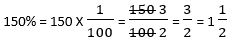## Conversion of Decimal into Percent

To convert a decimal number into percent, we must multiply the decimal number by 100 and write % sign along with it.

Example 1. Convert 0.96 into percent.

Solution.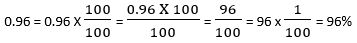Example 2. Convert 2.27 into percent.

Solution.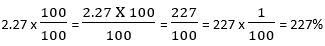## Conversion of Percent into Decimal

To convert a percent into decimal, we must move the decimal point two places to the left and take off the % sign.

Example 1. Convert 4% into decimal.

Solution.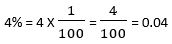Example 2. Convert 87% into decimal.

Solution.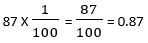Example 3. Convert 329% into decimal.

Solution.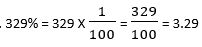## To Find Percentage of Given Quantity

To find a percent of given quantity, we must multiply the quantity by the equivalent fraction of the given percent.

Example 1. Find 80% of 650 marks.

Solution. 80% of 650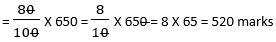Example 2. Find 20 % of 1000 kg.

Solution. 20% of 1000## Expressing One Quantity as Percent of Another

To express one quantity as a percent of another, we must convert both the quantities into the same units and then we must divide the given quantity to be expressed as percent of another quantity and multiply the result by 100.

Example 1. Express 525 grams as percent of 1250 grams.

Solution.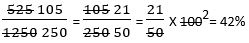Example 2. Express 45 cents as a percent of \$ 5.

Solution. 1 Dollar = 100 Cents

5 Dollars = 500 Cents

Now, the required percent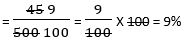## To Find a Quantity When a Given Percent of it is known

To find a quantity when a given percent of it is known, we must divide the known quantity by the given percentage.

Example 1. 25% of what volume is 500 ml?

Solution. 25% x Total Volume = 500 ml

Total Volume = 500 ml ÷ 25%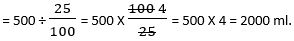So, 25% of 2000 ml is 500 ml.

## Percentage Test

Percentage Test - 1

Percentage Test - 2

## Class-5 Percentage Worksheet

Percentage Worksheet - 1

Percentage Worksheet - 2

Percentage Worksheet - 3

Percentage Worksheet - 4

Percentage Worksheet - 5

## Answer Sheet

Percentage-AnswerDownload the pdf

Copyright © 2021 LetsPlayMaths.com. All Rights Reserved.